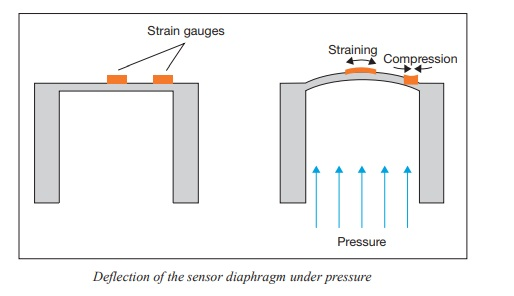# Electronic pressure measurement## Introduction

For electronic pressure measurement a sensor is required to detect the pressure and/or its change, and to convert it accurately and repeatably into an electrical signal utilising a physical operating principle. The electrical signal
is then a measure of the magnitude of the applied pressure or change in pressure. Four key measuring principles and their technical realization are shown below

## Resistive pressure measurement

The principle of resistive pressure measurement is based on the measurement of change in resistance of electrical conductors caused by a deflection dependent on pressure. The following equation applies to the resistance of an electrical conductor:

R=ρl/A

R Electrical resistance
ρ Resistivity
l Length
A Cross-sectional area

If a tensile force is applied to the conductor, its length increases and its cross-sectional area decreases (Fig. shown below). Since the resistivity of a metallic conductor is a (temperature-dependent) constant for a particular material and, therefore, independent of the geometry, the electrical resistance increases as a result of the elongation. In the case of compression, the opposite applies.The principle of resistive pressure measurement is realised using a main body which exhibits a controlled deflection under pressure.This main body frequently has a (thin) area referred to as the diaphragm, which is weakened intentionally. The degree of deflection caused by the pressure is measured using metallic strain gauges.

### Diaphragm with metallic strain gauges

Usually four strain gauges are applied to a diaphragm. Some of them are located on elongated and others on compressed areas of the diaphragm. If the diaphragm deflects under the action of a pressure, the strain gauges are deflected correspondingly (Fig. shown below). The electrical resistance increases or decreases proportionally to the deflection (elongation or compression). To accurately measure the resistance change, the strain gauges are wired to a Wheatstone measuring bridge.## Piezo-resistive pressure measurement

The principle of piezo-resistive pressure measurement is similar to the principle of resistive pressure measurement. However, since the strain gauges used for this measuring principle are made of a semiconductor material, their deflection due to elongation or compression results primarily in a change in resistivity. According to equation  (see above), the electrical resistance is proportional to the resistivity. While the piezo-resistive effect in metals is negligible and thus effectively insignificant within resistive pressure measurement, in semiconductors such as silicon it exceeds the effect of the variation of length and cross-section by a factor between 10 and 100

Silicon diaphragm with integrated strain gauges

Unlike metallic strain gauges, which can be attached to nearly any material, the semiconductor strain gauges are integrated into the diaphragm as microstructures. Thus, the strain gauges and the deflection body are based on the same semiconductor material. Usually four strain gauges are integrated into a diaphragm made of silicon and wired to a Wheatstone measuring bridge.

Encapsulation of the sensor element

Since the microstructures are not resistant to many pressure media, for most applications the sensor chip must be encapsulated. The pressure must then be transmitted indirectly to the semiconductor sensor element, e.g. using a
metallic diaphragm and oil as a transmission medium. Due to the magnitude of the piezo-resistive effect, piezo-resistive sensors can also be used in very low pressure ranges. However, due to strong temperature dependency and
manufacturing process-related variation, individual temperature compensation of every single sensor is required.

## Capacitive pressure measurement

The principle of capacitive pressure measurement is based on the measurement of the capacitance of a capacitor, which is dependent upon the plate separation. The capacitance of a dual-plate capacitor is determined using the following equation:

C = εA/d

C Capacitance of the dual-plate capacitor
ε Permittivity
A Area of the capacitor plate
d Plate separation

The principle of capacitive pressure measurement is realised using a main body with a metallic diaphragm, or one coated with a conductive material, which forms one of the two plates of a dual-plate capacitor. If the diaphragm
is deflected under pressure, the plate separation of the capacitor decreases, which results in an increase in its capacitance while the plates  surface area and permittivity remain constant (Fig. below).In this way, the pressure can be measured with high sensitivity. Therefore, capacitive pressure measurement is also suitable for very low pressure values, even down in the one-digit millibar range. The fact that the moving diaphragm
can be deflected until it reaches the fixed plate of the capacitor ensures a high overload safety for these pressure sensors. Practical restrictions on these sensors arise from the diaphragm material and its characteristics, and also from the required joining and sealing techniques.

### Piezo-electric pressure measurement

The principle of piezo-electric pressure measurement is based on the physical effect of the same name, only found in some non-conductive crystals, e.g. monocrystalline quartz. If such a crystal is exposed to pressure or tensile
force in a defined direction, certain opposed surfaces of the crystal are charged, positive and negative, respectively. Due to a displacement in the electrically charged lattice elements, an electric dipole moment results which is indicated by the (measurable) surface charges (Fig. below). The charge quantity is proportional to the value of the force, its polarity depends on the force direction. Electrical voltage created by the surface charges can be measured and amplifiedThe piezo-electric effect is only suitable for the measurement of dynamic pressures. In practice, piezo-electric pressure measurement is therefore restricted to specialised applications.